Home
Hostname: page-component-5c569c448b-ph4cd Total loading time: 0.226 Render date: 2022-07-05T12:45:50.520Z Has data issue: true Feature Flags: { "shouldUseShareProductTool": true, "shouldUseHypothesis": true, "isUnsiloEnabled": true, "useRatesEcommerce": false, "useNewApi": true } hasContentIssue true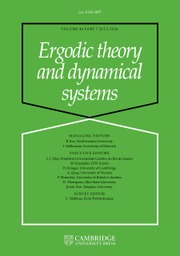Ergodic Theory and Dynamical Systems

## Abstract

We consider the dynamical system given by an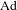$\text{Ad}$-diagonalizable element$a$ of the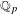$\mathbb{Q}_{p}$-points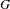$G$ of a unimodular linear algebraic group acting by translation on a finite volume quotient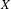$X$. Assuming that this action is exponentially mixing (e.g. if$G$ is simple) we give an effective version (in terms of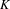$K$-finite vectors of the regular representation) of the following statement: If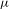${\it\mu}$ is an$a$-invariant probability measure with measure-theoretical entropy close to the topological entropy of$a$, then${\it\mu}$ is close to the unique$G$-invariant probability measure of$X$.

Type
Research Article
Information
Ergodic Theory and Dynamical Systems , September 2016 , pp. 1972 - 1988

## Access options

Get access to the full version of this content by using one of the access options below. (Log in options will check for institutional or personal access. Content may require purchase if you do not have access.)

## References

Adler, R. L. and Weiss, B.. Entropy, a complete metric invariant for automorphisms of the torus. Proc. Natl Acad. Sci. USA 57 (1967), 15731576.CrossRefGoogle Scholar
Bowen, R.. Entropy for group endomorphisms and homogeneous spaces. Trans. Amer. Math. Soc. 153 (1971), 401414.CrossRefGoogle Scholar
Borel, A. and Wallach, N.. Continuous Cohomology, Discrete Subgroups, and Representations of Reductive Groups (Mathematical Surveys and Monographs, 67) , 2nd edn. American Mathematical Society, Providence, RI, 2000.CrossRefGoogle Scholar
Cowling, M., Haagerup, U. and Howe, R.. Almost L 2 matrix coefficients. J. Reine Angew. Math. 387 (1988), 97110.Google Scholar
Cowling, M.. Sur les coefficients des représentations unitaires des groupes de Lie simples. Analyse Harmonique sur les Groupes de Lie (Sém., Nancy-Strasbourg 1976–1978, II) (Lecture Notes in Mathematics, 739) . Springer, Berlin, 1979, pp. 132178.Google Scholar
Cover, T. M. and Thomas, J. A.. Elements of Information Theory (Wiley Series in Telecommunications) . Wiley, New York, 1991.CrossRefGoogle Scholar
Einsiedler, M., Katok, A. and Lindenstrauss, E.. Invariant measures and the set of exceptions to Littlewood’s conjecture. Ann. of Math. (2) 164(2) (2006), 513560.CrossRefGoogle Scholar
Einsiedler, M. and Lindenstrauss, E.. Diagonal actions on locally homogeneous spaces. Homogeneous Flows, Moduli Spaces and Arithmetic (Clay Mathematics Monographs, 10) . American Mathematical Society, Providence, RI, 2010, pp. 155241.Google Scholar
Einsiedler, M. and Ward, T.. Ergodic Theory with a View Towards Number Theory (Graduate Texts in Mathematics, 259) . Springer, London, 2011.Google Scholar
Howe, R. E. and Moore, C. C.. Asymptotic properties of unitary representations. J. Funct. Anal. 32(1) (1979), 7296.CrossRefGoogle Scholar
Howe, R. and Tan, E.-C.. Non-Abelian Harmonic Analysis: Applications of SL (2, R) (Universitext) . Springer, New York, 1992.CrossRefGoogle Scholar
Kadyrov, S.. Effective uniqueness of Parry measure and exceptional sets in ergodic theory. Monatsh. Math. (2014), to appear, doi:10.1007/s00605-014-0690-7.Google Scholar
Margulis, G. A.. Discrete Subgroups of Semisimple Lie Groups (Ergebnisse der Mathematik und ihrer Grenzgebiete (3) [Results in Mathematics and Related Areas (3)], 17) . Springer, Berlin, 1991.CrossRefGoogle Scholar
Margulis, G. A. and Tomanov, G. M.. Invariant measures for actions of unipotent groups over local fields on homogeneous spaces. Invent. Math. 116(1–3) (1994), 347392.CrossRefGoogle Scholar
Oh, H.. Uniform pointwise bounds for matrix coefficients of unitary representations and applications to Kazhdan constants. Duke Math. J. 113(1) (2002), 133192.CrossRefGoogle Scholar
Polo, F.. Equidistribution in chaotic dynamical systems. PhD Thesis, The Ohio State University, 2011.Google Scholar
Platonov, V. and Rapinchuk, A.. Algebraic Groups and Number Theory (Pure and Applied Mathematics, 139) . Academic Press, Boston, MA, 1994; translated from the 1991 Russian original by Rachel Rowen.Google Scholar
Ratner, M.. On the p-adic and S-arithmetic generalizations of Raghunathan’s conjectures. Lie Groups and Ergodic Theory (Mumbai, 1996) (Tata Institute of Fundamental Research Studies in Mathematics, 14) . Tata Institute of Fundamental Research, Bombay, 1998, pp. 167202.Google Scholar
Serre, J.-P.. Lie Algebras and Lie Groups: 1964 Lectures given at Harvard University (Lecture Notes in Mathematics, 1500) , 2nd edn. Springer, Berlin, 1992.Google Scholar
Shalom, Y.. Rigidity, unitary representations of semisimple groups, and fundamental groups of manifolds with rank one transformation group. Ann. of Math. (2) 152(1) (2000), 113182.CrossRefGoogle Scholar
Silberger, A. J.. Introduction to Harmonic Analysis on Reductive p-adic Groups (Mathematical Notes, 23) . Princeton University Press, Princeton, NJ, 1979, pp. 19711973; based on lectures by Harish-Chandra at the Institute for Advanced Study.Google Scholar
4
Cited by

# Save article to Kindle

Note you can select to save to either the @free.kindle.com or @kindle.com variations. ‘@free.kindle.com’ emails are free but can only be saved to your device when it is connected to wi-fi. ‘@kindle.com’ emails can be delivered even when you are not connected to wi-fi, but note that service fees apply.

Find out more about the Kindle Personal Document Service.

Effectivity of uniqueness of the maximal entropy measure on$p$-adic homogeneous spaces Available formats × # Save article to Dropbox To save this article to your Dropbox account, please select one or more formats and confirm that you agree to abide by our usage policies. If this is the first time you used this feature, you will be asked to authorise Cambridge Core to connect with your Dropbox account. Find out more about saving content to Dropbox. Effectivity of uniqueness of the maximal entropy measure on$p$-adic homogeneous spaces
Available formats
×

# Save article to Google Drive

To save this article to your Google Drive account, please select one or more formats and confirm that you agree to abide by our usage policies. If this is the first time you used this feature, you will be asked to authorise Cambridge Core to connect with your Google Drive account. Find out more about saving content to Google Drive.

Effectivity of uniqueness of the maximal entropy measure on$p\$-adic homogeneous spaces
Available formats
×
×